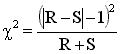## Example. Analyze matched case-control study.

This example (adapted from Intuitive Biostatistics ) helps you use the McNemar's test calculator that is part of GraphPad QuickCalcs. Go back to it.

In a standard case-control study, the investigator compares a group of controls with a group of cases. As a group, the controls are supposed to be similar to the cases (except for the absence of disease). Another way to perform a case-control study is to match individual cases with individual controls based on age, gender, occupation, location and other relevant variables.

Displaying and analyzing data from matched case-control studies on an ordinary contingency table obscures the fact that the cases and controls were matched. Matching makes the experiment stronger, so the analysis ought to take it into account. Here are some sample data:

 Control + - Total Case + 13 25 38 - 4 92 96 Total 17 117 134

The investigators studied 134 cases and 134 matched controls, for a total of 268 subjects. Each entry in the table represents one pair (a case and a control). This is not a contingency table, so the usual analyses of contingency tables would not be helpful. It turns out that the odds ratio can be computed quite simply. The 13 pairs in which both cases and controls were exposed to the risk factor provide no information about the association between risk factor and disease. Similarly, the 92 pairs in which neither case nor control were exposed to risk factor provide no information. The odds ratio is calculated as the ratio of the other two values: pairs in which the case was exposed to the risk factor but the control was not divided by pairs in the control was exposed to the risk factor but the case was not. In this example, the odds ratio for the association between risk factor and disease is 25/4 = 6.25. The equation for the confidence interval is complicated (see page 286 of S. Selvin, Statistical Analysis of Epidemiologic Data, 2nd edition ). The 95% confidence interval for the odds ratio ranges from 2.158 to 24.710. ( Intuitive Biostatistics gives a simple approximate equation, so the confidence interval is a bit different).

You can interpret the odds ratio from a matched case-control study just as you would interpret the odds ratio from an ordinary case-control study. If we assume that the disease is fairly rare, then we can conclude that exposure to the risk factor increases ones' risk 6.25 fold.

McNemar's test calculates a P value. This test uses only the number of discordant pairs, that is, the number of pairs for which the control was exposed to the risk factor but the case was not (4 in this example) and the number of pairs where the case was exposed to risk factor but the control was not (25 in this example). Call these two numbers R and S. Calculate chi-square using this equation:For this example, chi-square=13.79, which has one degree of freedom. The two-tailed P value is 0.0002. If there were really no association between risk factor and disease, there is a 0.02 percent chance that the observed odds ratio would be so far from 1.0 (no association).

## Using GraphPad's McNemar test calculator

Enter the data into GraphPad's calculator like this:
 Risk Factor? Control Case # of pairs No Yes 25 Yes No 4 Yes Yes 13 No No 92

Here are the results:

 Summary: If there were no association between the risk factor and the disease, you'd expect the number of pairs where cases was exposed to the risk factor but control was not to equal the number of pairs where the control was exposed to the risk factor but the case did not. In this study, there were 29 discordant pairs (case and control had different exposure to the risk factor). There were 25 ( 86.207%) pairs where the control was exposed to the risk factor but the case was not, and 4 ( 13.793%) pairs where the case was exposed to the risk factor but the control was not. P Value: The two-tailed P value equals 0.0002 By conventional criteria, this difference is considered to be extremely statistically significant. The P value was calculated with McNemar's test with the continuity correction. Chi squared equals 13.793 with 1 degrees of freedom. The P value answers this question: If there is no association between risk factor and disease, what is the probability of observing such a large discrepancy (or larger) between the number of the two kinds of discordant pairs? A small P value is evidence that there is an association between risk factor and disease. Odds ratio: The odds ratio is 6.250, with a 95% confidence interval extending from 2.158 to 24.710